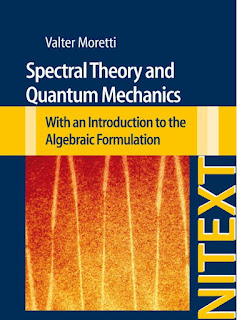CONCEPTS OF PHYSICS

# Spectral Theory and Quantum Mechanics; with an Introduction to the Algebraic Formulation PDF Free DownloadThis book discusses the mathematical foundations of quantum theories. It offers an introductory text on linear functional analysis with a focus on Hilbert spaces, highlighting the spectral theory features that are relevant in physics. After exploring physical phenomenology, it then turns its attention to the formal and logical aspects of the theory. Further, this Second Edition collects in one volume a number of useful rigorous results on the mathematical structure of quantum mechanics focusing in particular on von Neumann algebras, Super selection rules, the various notions of Quantum Symmetry and Symmetry Groups, and including a number of fundamental results on the algebraic formulation of quantum theories.